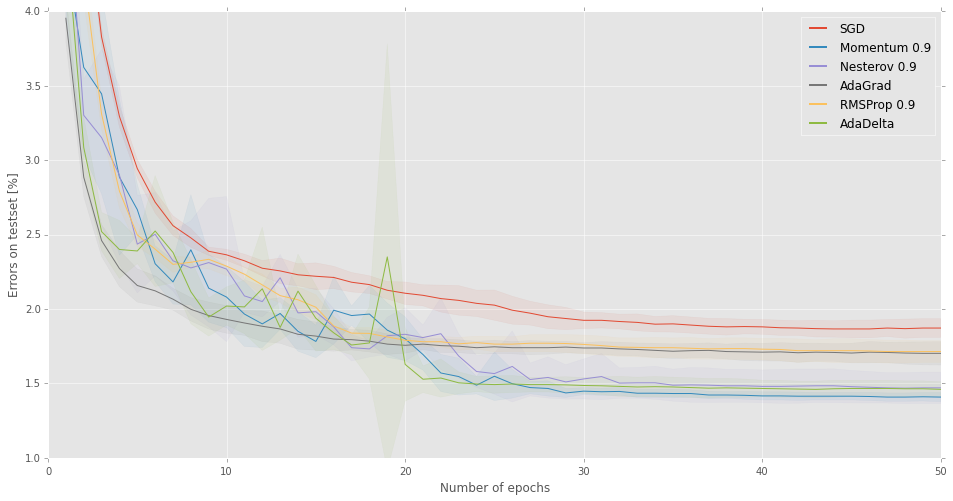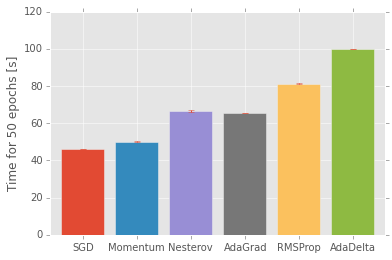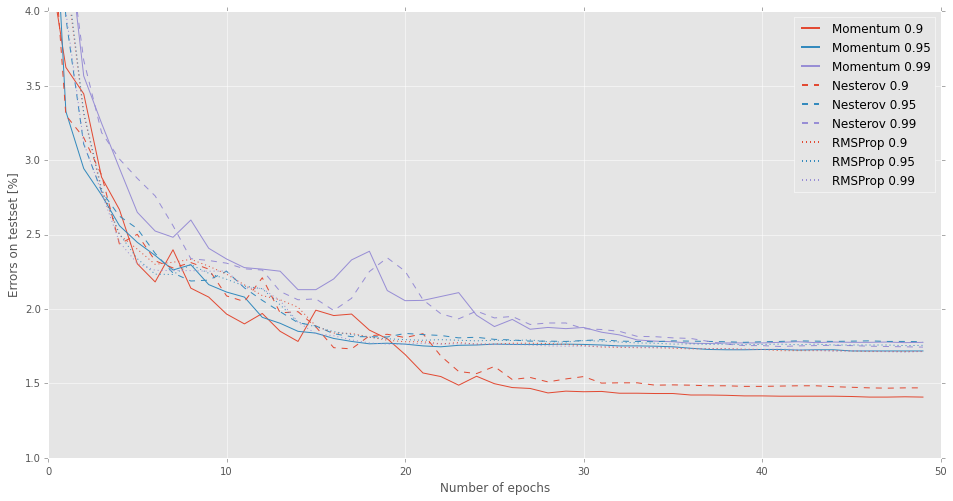This notebook is A) an attempt to reproduce the plots in Matt Zeiler's AdaDelta tech report and B) investigate AdaDelta and various other first-order optimization methods beyond what Matt did in his report.

I will be using the DeepFried Theano library/toolbox for this.

In :
import numpy as np
import matplotlib.pyplot as plt
import sys
import time
from IPython.display import HTML, display
from IPython.html import widgets

In :
%matplotlib inline

# Font which got unicode math stuff.
import matplotlib as mpl
mpl.rcParams['font.family'] = 'DejaVu Sans'

plt.style.use('ggplot')

In :
import DeepFried as df

Using gpu device 0: GeForce GTX 980


# Utility functions¶

You can skip reading these functions. They will be used mostly for prettier presentation of the results and are —I think— self-explanatory boilerplate code.

In :
def printnow(where, fmt, *a, **kw):
if where is not None:
where.write(fmt.format(*a, **kw))
where.flush()

In :
def make_table(data, header, margin, datafmt='{:.2%}', aggfn=None):
if aggfn is None:
aggfn = lambda data: (datafmt + ' ± ' + datafmt).format(np.mean(data), np.std(data))

s = '<table><tr><th>'
s += '<th style="text-align: center">' + h
for m, row in zip(margin, data):
s += '<tr><th>' + m
for d in row:
s += '<td style="text-align: center">' + datafmt.format(d)
if data.ndim > 1 and aggfn is not False:
s += '<th>' + aggfn(row)
s += '</tr>'
if data.ndim > 1 and aggfn is not False:
s += '<tr><th>'
for col in data.T:
s += '<th style="text-align: center">' + aggfn(col)
s += '</table>'
return HTML(s)

In :
def plot_banded_error(errs, label, ax=None):
ax = ax or plt
m = np.mean(errs, axis=0)*100
s = np.std(errs, axis=0)*100
line, = ax.plot(np.arange(1, len(m)+1), m, label=label)
ax.fill_between(np.arange(1, len(m)+1), m-s, m+s, color=line.get_color(), alpha=0.075)

In :
def fatlegend(ax, *a, **kw):
leg = ax.legend(*a, **kw)
for l in leg.legendHandles:
l.set_linewidth(l.get_linewidth()*2.0)
return leg


In :
import pickle
import gzip
(Xtr, ytr), (Xva, yva), (Xte, yte) = pickle.load(gzip.open('mnist.pkl.gz'), encoding='latin1')
ytr, yva, yte = ytr.astype(np.int32), yva.astype(np.int32), yte.astype(np.int32)
Xtr.shape, Xva.shape, Xte.shape

Out:
((50000, 784), (10000, 784), (10000, 784))

The paper compares experiments to the No More Pesky Learning Rates paper (referenced to as "pesky" from now on), which talks about a 60k training-set, i.e. they merged training and validation. Let's do this too:

In :
X, y = np.concatenate((Xtr, Xva)), np.concatenate((ytr, yva))
X.shape, y.shape

Out:
((60000, 784), (60000,))

# Build the paper's Deep Tanh Network¶

The paper's description of the (first) model:

For comparison with Schaul et al.'s method we trained with tanh nonlinearities and 500 hidden units in the first layer followed by 300 hidden units in the second layer, with the final softmax output layer on top. Our method was trained on mini-batches of 100 images per batch for 6 epochs through the training set.

This is a very standard architecture which is easily built using the Sequence container in, technically, a single line of code:

In :
tanh_model = df.containers.Sequence(
df.layers.FullyConnected(28*28, 500),
df.layers.Tanh(init=0.09),
df.layers.FullyConnected(500, 300),
df.layers.Tanh(init=0.09),
df.layers.FullyConnected(300, 10),
df.layers.Softmax()
)


Now we need to pass that model to the AdaDelta optimizer along with a cost that should be minimized. The optimizer will "compile" AdaDelta's update-function for this specific model and cost. The paper further details the used training hyperparameters as

Setting the hyperparameters to $\epsilon$ = 1e − 6 and $\rho$ = 0.95 we achieve 2.00% test set error compared to the 2.10% of Schaul et al.

Note that this implementation of the optimizer actually uses the paper's values as default values for these hyperparameters, so we wouldn't even need to explicitly set them here.

In :
tanh_model_adadelta = df.optim.StreaMiniAdaDelta(100, tanh_model, df.costs.CategoricalCrossEntropy(), rho=0.95, eps=1e-6)


Before starting learning, I need to (re-)initialize both the model and the optimizer. The former will have its weights set to random initial values using the given random seed $13$ according to the initialization parameters of the nonlinearities, while the latter will have its accumulators for momentum-like quantities zeroed. All parameters are initialized to NaN by default, so that there'll be a lot of noise in case one forgets about initialization.

In :
tanh_model.reinit(rng=13)


Finally, as stated in the above quote, the model is trained for only six epochs, so we can simply iterate:

In :
for epoch in range(6):
printnow(sys.stdout, "Training epoch {} ... \n", epoch+1)

Training epoch 1 ...
Training epoch 2 ...
Training epoch 3 ...
Training epoch 4 ...
Training epoch 5 ...
Training epoch 6 ...


Now let's look at the error on the test set; for doing so we need a Predictor which compiles the code for doing prediction. We can then use the predictions to compute the error, which should be around 2.00%.

In :
tanh_model_pred = df.pred.StreaMiniPredictor(100, tanh_model)

In :
print("Error: {:.2%}".format(df.postproc.classification_error(tanh_model_pred.pred_epoch(Xte), yte, percent=True)))

Error: 2.02%


This looks pretty good.

# The importance of initialization and seeds¶

While the above looks good and seems to reproduce the note's results, I deliberately chose an initialization with a standard deviation of $\sigma = 0.09$ and a random seed of $r = 13$. Let's run a number of experiments with different seeds and with slightly different initializations.

In :
def init_seed_experiment(stds, seeds, nepochs=6):
errors = np.empty((len(stds), len(seeds)))
progress = widgets.IntProgress(value=0, min=0, max=len(stds)*len(seeds)-1, description='Training:')
display(progress)
for i, std in enumerate(stds):
model = df.containers.Sequence(
df.layers.FullyConnected(28*28, 500),
df.layers.Tanh(init=std),
df.layers.FullyConnected(500, 300),
df.layers.Tanh(init=std),
df.layers.FullyConnected(300, 10),
df.layers.Softmax()
)
pred = df.pred.StreaMiniPredictor(100, model)

for j, r in enumerate(seeds):
model.reinit(rng=r)

for epoch in range(nepochs):

errors[i,j] = df.postproc.classification_error(pred.pred_epoch(Xte), yte, percent=True)
progress.value += 1
return errors

In :
stds = (0.05, 0.08, 0.1)
seeds = (7, 13, 42)
errors = init_seed_experiment(stds, seeds)

In :
make_table(errors, ['r = ' + str(r) for r in seeds], ['σ = ' + str(s) for s in stds])

Out:
r = 7r = 13r = 42
σ = 0.052.31%2.28%2.25%2.28% ± 0.02%
σ = 0.082.12%1.98%2.14%2.08% ± 0.07%
σ = 0.12.22%2.03%2.31%2.19% ± 0.12%
2.22% ± 0.08%2.10% ± 0.13%2.23% ± 0.07%

From this preliminary experiment, it looks like the seed influences the error-rate just as much as the initialization, though that might be due to either too early stopping or too few seeds tried, i.e. luck. Let's first investigate the former by re-running the exact same experiment for a much larger number of epochs: 50. This seems like a reasonable count given the error-curves displayed in Fig.1 of the report.

In :
errors50 = init_seed_experiment(stds, seeds, nepochs=50)

.........
In :
make_table(errors50, ['r = ' + str(r) for r in seeds], ['σ = ' + str(s) for s in stds])

Out:
r = 7r = 13r = 42
σ = 0.051.81%1.78%1.78%1.79% ± 0.01%
σ = 0.081.83%1.72%1.77%1.77% ± 0.04%
σ = 0.11.95%1.66%1.88%1.83% ± 0.12%
1.86% ± 0.06%1.72% ± 0.05%1.81% ± 0.05%

While running for a longer time imporves things a bit, There's still as large as $0.3$ percentage points difference between different seeds, which is three times as much as the $0.1\%$ difference between $2.00\%$ and $2.10\%$ pointed at in the report. Just to confirm that a difference of $0.1\%$ is not really significant for 6 epochs, let's run 6 epochs with much more seeds, so that we get a good estimate of the standard deviation:

In :
manyseeds = np.random.randint(2**31, size=20)
errors_manyseeds = init_seed_experiment(stds + ('Xavier',), manyseeds, nepochs=6)

In :
mean = np.mean(errors_manyseeds, axis=1)
std = np.std(errors_manyseeds, axis=1)
ptp = np.ptp(errors_manyseeds, axis=1)
make_table(np.vstack((mean, std, ptp)).T,
['mean', 'std. dev.', 'max - min'], ['σ = ' + str(s) for s in stds] + ['Xavier'], aggfn=False)

Out:
meanstd. dev.max - min
σ = 0.052.30%0.08%0.30%
σ = 0.082.22%0.12%0.41%
σ = 0.12.24%0.12%0.39%
Xavier2.21%0.08%0.38%

Yep, we can clearly see that $0.1\%$ is well within the variance due to the chosen random seed, thus we shall not make any conclusions based on that figure. Matt doesn't really do so anyways in his report, stating that

While this is nowhere near convergence it gives a sense of how quickly the algorithms can optimize the classification objective.

I simply want to stress that it is important A) to report the used initialization, which is unfortunately left unspecified throughout the report, as well as B) to run multiple experiments with a varying seed in order to determine the significance of a result.

Regarding A), I will simply use Xavier initialization for the remainder of this notebook as it is nowaday's go-to choice for initialization and it performs in the same ballpark as other initializations as seen in the previous experiment.

Regarding B), I will run each experiment five times with a random seed each time and always report mean and standard deviation of all experiments. This way, we can see whether a difference between two experiments is significant or not.

Let's now move on to the meat.

# The Deep Rectified Network¶

For the main part of the experiments, Rectified Linear Units (ReLUs) are used instead of tanh nonlinearities, everything else stays the same. Xavier initialization is the default initialization used by df.layers.ReLU and we thus don't need to specify anything.

In :
relu_model = df.containers.Sequence(
df.layers.FullyConnected(28*28, 500),
df.layers.ReLU(),
df.layers.FullyConnected(500, 300),
df.layers.ReLU(),
df.layers.FullyConnected(300, 10),
df.layers.Softmax()
)


## Table 2: Robustness to hyperparameters¶

Table 2 lists the test error on MNIST after training for just 6 epochs with various values for the parameters $\rho$ and $\epsilon$. The aim of this table is to show that AdaDelta is not very sensitive to its two hyperparameters.

In :
def table2_experiment(model, rho, eps, nrounds=5, nepochs=6):
pred = df.pred.StreaMiniPredictor(100, model)

errors = np.empty((len(eps), len(rho), nrounds))

progress = widgets.IntProgress(value=0, min=0, max=np.prod(errors.shape)*nepochs-1, description='Experiments:')
display(progress)

for i, e in enumerate(eps):
for j, r in enumerate(rho):
for k in range(nrounds):
model.reinit(None) ; optim.reinit()
for epoch in range(nepochs):
optim.fit_epoch(X, y)
progress.value += 1
errors[i,j,k] = df.postproc.classification_error(pred.pred_epoch(Xte), yte, percent=True)

return errors

rhos = (0.9, 0.95, 0.99)
epss = (1e-2, 1e-4, 1e-6, 1e-8)
table2_errors = table2_experiment(relu_model, rhos, epss)

In :
make_table(np.concatenate((np.mean(table2_errors, axis=2)[:,:,np.newaxis],
np.std(table2_errors, axis=2)[:,:,np.newaxis]), axis=2),
['ρ = ' + str(r) for r in rhos], ['ϵ = {:.0e}'.format(e) for e in epss],
datafmt='{0:.2%} ± {0:.2%}', aggfn=False)

Out:
ρ = 0.9ρ = 0.95ρ = 0.99
ϵ = 1e-022.69% ± 0.19%2.99% ± 0.77%3.14% ± 0.30%
ϵ = 1e-042.48% ± 0.39%2.32% ± 0.15%2.41% ± 0.14%
ϵ = 1e-062.18% ± 0.09%2.15% ± 0.09%2.29% ± 0.35%
ϵ = 1e-082.63% ± 0.04%2.45% ± 0.06%2.16% ± 0.04%

Qualitatively, this table is the same as in the report: the lowest error is reached using $\rho=0.95$ and $\epsilon = 1e^{-6}$, and the errors slightly increase but don't get too large when modifying the hyperparameters. Quantitatively though, the whole table's average error is rougly $0.3\%$ higher than in the AdaDelta report. I'm not sure if this difference stems from the initialization I've used, a bug in my code, or if I'm missing something else. If anybody finds out, please let me know.

Just for reference, here's the same table when taking the lowest error across the five runs:

In :
make_table(np.min(table2_errors, axis=2),
['ρ = ' + str(r) for r in rhos], ['ϵ = {:.0e}'.format(e) for e in epss], aggfn=False)

Out:
ρ = 0.9ρ = 0.95ρ = 0.99
ϵ = 1e-022.41%2.37%2.70%
ϵ = 1e-042.14%2.14%2.20%
ϵ = 1e-062.09%2.02%1.88%
ϵ = 1e-082.57%2.38%2.11%

The aim of this table is to show that other first-order optimization methods that are frequently used are much more sensitive to their hyperparameters than AdaDelta. I will make this experiment a little more exhaustive by adding all other optimization methods which are implemented in DeepFried, as well as run with multiple values of other methods' additional hyperparameters. This experiment will take quite some time, as it's runing $5 \cdot 11 \cdot 5 = 275$ trainings of six epochs, totalling $1650$ epochs; rejoice of the Jupyter progressbar widget!

In :
from collections import OrderedDict

def table1_experiment(model, lrs, optims, nrounds=5, nepochs=6):
pred = df.pred.StreaMiniPredictor(100, model)

errors = np.empty((len(lrs), len(optims), nrounds))

progress = widgets.IntProgress(value=0, min=0, max=np.prod(errors.shape)*nepochs-1, description='Experiments:')
display(progress)

for i, lr in enumerate(lrs):
for j, optim in enumerate(optims):
for k in range(nrounds):
model.reinit(None) ; optim.reinit()
for epoch in range(nepochs):
optim.fit_epoch(X, y, lrate=lr)
progress.value += 1
errors[i,j,k] = df.postproc.classification_error(pred.pred_epoch(Xte), yte, percent=True)

return errors

cost = df.costs.CategoricalCrossEntropy()
lrs = (1e0, 1e-1, 1e-2, 1e-3, 1e-4)
optims = OrderedDict((('SGD', df.optim.StreaMiniSGD(100, relu_model, cost)),
('Momentum .9', df.optim.StreaMiniMomentum(100, relu_model, cost, momentum=0.9 , nesterov=False)),
('Momentum .95', df.optim.StreaMiniMomentum(100, relu_model, cost, momentum=0.95, nesterov=False)),
('Momentum .99', df.optim.StreaMiniMomentum(100, relu_model, cost, momentum=0.99, nesterov=False)),
('Nesterov .9', df.optim.StreaMiniMomentum(100, relu_model, cost, momentum=0.9 , nesterov=True)),
('Nesterov .95', df.optim.StreaMiniMomentum(100, relu_model, cost, momentum=0.95, nesterov=True)),
('Nesterov .99', df.optim.StreaMiniMomentum(100, relu_model, cost, momentum=0.99, nesterov=True)),
('RMSProp .9', df.optim.StreaMiniRMSProp(100, relu_model, cost, 0.9)),
('RMSProp .95', df.optim.StreaMiniRMSProp(100, relu_model, cost, 0.95)),
('RMSProp .99', df.optim.StreaMiniRMSProp(100, relu_model, cost, 0.99))))
table1_errors = table1_experiment(relu_model, lrs, optims.values(), nrounds=5, nepochs=6)

In :
make_table(np.concatenate((np.mean(table1_errors, axis=2)[:,:,np.newaxis],
np.std(table1_errors, axis=2)[:,:,np.newaxis]), axis=2),
optims.keys(), ['λ₀={:.0e}'.format(l) for l in lrs],
datafmt='{0:.2%} ±{0:.2%}', aggfn=False)

Out:
λ₀=1e+0037.89% ±42.71%89.68% ±0.00%89.78% ±0.21%90.21% ±0.02%89.68% ±0.00%89.68% ±0.00%89.68% ±0.00%60.29% ±14.15%89.91% ±0.00%90.25% ±0.00%89.34% ±1.80%
λ₀=1e-012.68% ±0.13%2.31% ±0.24%3.49% ±0.57%88.65% ±0.00%2.49% ±0.26%3.32% ±0.16%88.65% ±0.00%3.30% ±0.22%68.60% ±10.59%72.21% ±9.98%88.46% ±3.92%
λ₀=1e-027.09% ±0.12%2.74% ±0.05%2.47% ±0.07%3.12% ±0.24%2.73% ±0.11%2.53% ±0.08%2.82% ±0.21%2.07% ±0.06%3.01% ±0.48%3.07% ±0.20%3.47% ±0.22%
λ₀=1e-0317.41% ±0.50%6.99% ±0.17%5.37% ±0.11%2.55% ±0.14%6.97% ±0.11%5.34% ±0.10%2.72% ±0.03%6.82% ±0.11%2.37% ±0.16%2.42% ±0.13%2.33% ±0.04%
λ₀=1e-0453.38% ±7.07%17.61% ±0.51%12.33% ±0.23%7.04% ±0.08%17.26% ±0.66%12.19% ±0.29%7.06% ±0.09%19.93% ±0.86%6.66% ±0.16%6.64% ±0.09%6.40% ±0.09%

This confirms the report's point that other optimization algorithms have a very tight sweet spot for their optimal parameter values, although it's not that bad: all typically used momentum "strenghts" give acceptable results for an order of magnitude values of the learning-rate.

# Figure 1: Error curves of various methods¶

Let's pick the best settings for each of the optimizers as found in the previous table and record their test-error throughout the epochs.

In :
def record_training(trainer, nrounds=5, nepochs=50, **kw):
pred = df.pred.StreaMiniPredictor(100, trainer.model)
errs = np.empty((nrounds,nepochs))
times = np.empty(nrounds)

progress = widgets.IntProgress(value=0, min=0, max=np.prod(errs.shape)-1, description='Experiments:')
display(progress)

for i in range(nrounds):
trainer.model.reinit(None) ; trainer.reinit()
tstart = time.clock()
for epoch in range(nepochs):
trainer.fit_epoch(X, y, **kw)
errs[i,epoch] = df.postproc.classification_error(pred.pred_epoch(Xte), yte, percent=True)
progress.value += 1
times[i] = time.clock() - tstart
return errs, times

In :
errs_sgd, t_sgd = record_training(df.optim.StreaMiniSGD(100, relu_model, cost), lrate=1e-1)
errs_mom1, t_mom1 = record_training(df.optim.StreaMiniMomentum(100, relu_model, cost, momentum=0.9 , nesterov=False), lrate=1e-1)
errs_mom2, t_mom2 = record_training(df.optim.StreaMiniMomentum(100, relu_model, cost, momentum=0.95, nesterov=False), lrate=1e-2)
errs_mom3, t_mom3 = record_training(df.optim.StreaMiniMomentum(100, relu_model, cost, momentum=0.99, nesterov=False), lrate=1e-3)
errs_nes1, t_nes1 = record_training(df.optim.StreaMiniMomentum(100, relu_model, cost, momentum=0.9 , nesterov=True), lrate=1e-1)
errs_nes2, t_nes2 = record_training(df.optim.StreaMiniMomentum(100, relu_model, cost, momentum=0.95, nesterov=True), lrate=1e-2)
errs_nes3, t_nes3 = record_training(df.optim.StreaMiniMomentum(100, relu_model, cost, momentum=0.99, nesterov=True), lrate=1e-3)
errs_rms1, t_rms1 = record_training(df.optim.StreaMiniRMSProp(100, relu_model, cost, 0.9), lrate=1e-3)
errs_rms2, t_rms2 = record_training(df.optim.StreaMiniRMSProp(100, relu_model, cost, 0.95), lrate=1e-3)
errs_rms3, t_rms3 = record_training(df.optim.StreaMiniRMSProp(100, relu_model, cost, 0.99), lrate=1e-3)

In :
fig, ax = plt.subplots(1,1, figsize=(16,8))
plot_banded_error(errs_sgd, label='SGD', ax=ax)
plot_banded_error(errs_mom1, label='Momentum 0.9', ax=ax)
plot_banded_error(errs_nes1, label='Nesterov 0.9', ax=ax)
plot_banded_error(errs_rms1, label='RMSProp 0.9', ax=ax)
ax.grid(True)
ax.set_ylim(1, 4)
ax.set_xlabel("Number of epochs")
ax.set_ylabel("Errors on testset [%]")
fatlegend(ax)

Out:
<matplotlib.legend.Legend at 0x7f65cd409b70>This plot is very similar to Figure 1 in the report, with most methods plateauing at $1.5\%$ error, momentum being marginally better than AdaDelta, and plain SGD being by far the worst optimizer.

# Going further¶

This is the point where I diverge from the report because I can't reproduce the distributed network part and there's some more things I want to investigate.

## Wall-clock times¶

There's more to an optimizer than the converged error rate: who'd want to wait for ages just to be a few percentage points better? If a method is much faster, that time can be spent looking for better architectures, augmentations, ... In the end, all we care about is wall-clock time $t_w$ to a good result. This wall-clock time can be divided into two parts: the number of epochs $n_e$ to a good result and the time spend per epoch $t_e$, such that $t_w = n_e \cdot t_e + \epsilon$, where $\epsilon$ is more-or-less irrelevant.

From the previous plot (representing Fig.1 from the report), we can see that for this simple network on this simple MNIST dataset, all methods have roughly converged after $n_e = 30$ epochs. I have so far never seen $t_e$ reported in a paper, so let's have a look at that one next.

In :
def plot_times(experiments):
N = len(experiments)
W = 0.8
margin = 1-W
plt.bar(np.arange(N), list(map(np.mean, experiments.values())), W, yerr=list(map(np.std, experiments.values())), color=mpl.rcParams['axes.color_cycle'])
plt.xticks(np.arange(N)+W/2, list(experiments.keys()))
plt.xlim(-margin, N-1+W+margin)
plt.ylabel("Time for 50 epochs [s]")

plot_times(OrderedDict((
("SGD", t_sgd),
("Momentum", np.concatenate((t_mom1, t_mom2, t_mom3))),
("Nesterov", np.concatenate((t_nes1, t_nes2, t_nes3))),
("RMSProp", np.concatenate((t_rms1, t_rms2, t_rms3))),
)There's two conclusions I see here:

1. Obviously, the more complex the optimizer, the slower it gets.
2. Plain momentum, if tuned properly, gives the most bang for the buck.

## The momentum parameter¶

I also ran all of the momentum-based methods (except for AdaDelta) with the three typically used momentum "strenghts:" $0.9$, $0.95$ and $0.99$ ; the following plot compares their training curves:

In :
fig, ax = plt.subplots(1,1, figsize=(16,8))
ax.plot(np.mean(errs_mom1, axis=0)*100, label='Momentum 0.9', color='#E24A33', ls='-')
ax.plot(np.mean(errs_mom2, axis=0)*100, label='Momentum 0.95', color='#348ABD', ls='-')
ax.plot(np.mean(errs_mom3, axis=0)*100, label='Momentum 0.99', color='#988ED5', ls='-')
ax.plot(np.mean(errs_nes1, axis=0)*100, label='Nesterov 0.9', color='#E24A33', ls='--')
ax.plot(np.mean(errs_nes2, axis=0)*100, label='Nesterov 0.95', color='#348ABD', ls='--')
ax.plot(np.mean(errs_nes3, axis=0)*100, label='Nesterov 0.99', color='#988ED5', ls='--')
ax.plot(np.mean(errs_rms1, axis=0)*100, label='RMSProp 0.9', color='#E24A33', ls=':')
ax.plot(np.mean(errs_rms2, axis=0)*100, label='RMSProp 0.95', color='#348ABD', ls=':')
ax.plot(np.mean(errs_rms3, axis=0)*100, label='RMSProp 0.99', color='#988ED5', ls=':')
ax.grid(True)
ax.set_ylim(1, 4)
ax.set_xlabel("Number of epochs")
ax.set_ylabel("Errors on testset [%]")
fatlegend(ax)

Out:
<matplotlib.legend.Legend at 0x7f65cda1a860>The conclusions I draw for this plot, again restricted to this small network on this dataset, is that RMSProp (the very lightly dotted lines) is pretty consistent across strength of momentum, while classical momentum and Nesterov momentum are consistent with eachother but vary widely across momentum strength.

# Future work¶

For this analysis to really be complete, I'd like to add following parts when I get the time to:

1. Analysis of the effective learning rates (Section 4.3 of the note). This should be relatively easy, since the accumulated quantities are readily available as sh_g2 and sh_delta2 attributes of the optimizer object.
2. Analysis of robustness on other datasets. For example, I had to dramatically change the value of $\epsilon$ for AdaDelta to work at all on the NSDB Kaggle challenge dataset. This suggests less practical robustness than what the report sells.